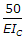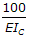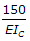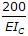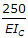# Civil Engineering - Theory of Structures

Exercise : Theory of Structures - Section 2
16.
The ratio of shear stress and shear strain of an elastic material, is
Modulus of Rigidity
Shear Modulus
Young's Modulus
Modulus of Elasticity
both (a) and (b).
Explanation:
No answer description is available. Let's discuss.

17.
The ratio of the maximum deflections of a simply supported beam with a central load W and of a cantilever of same length and with a load W at its free end, is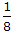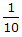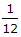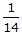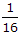Explanation:
No answer description is available. Let's discuss.

18.
The greatest load which a spring can carry without getting permanently distorted, is called
stiffness
prof resilience
proof stress
Explanation:
No answer description is available. Let's discuss.

19.
The horizontal deflection of a parabolic curved beam of span 10 m and rise 3 m when loaded with a uniformly distributed load l t per horizontal length, is (where Ic is the M.I. at the crown, which varies as the slope of the arch).